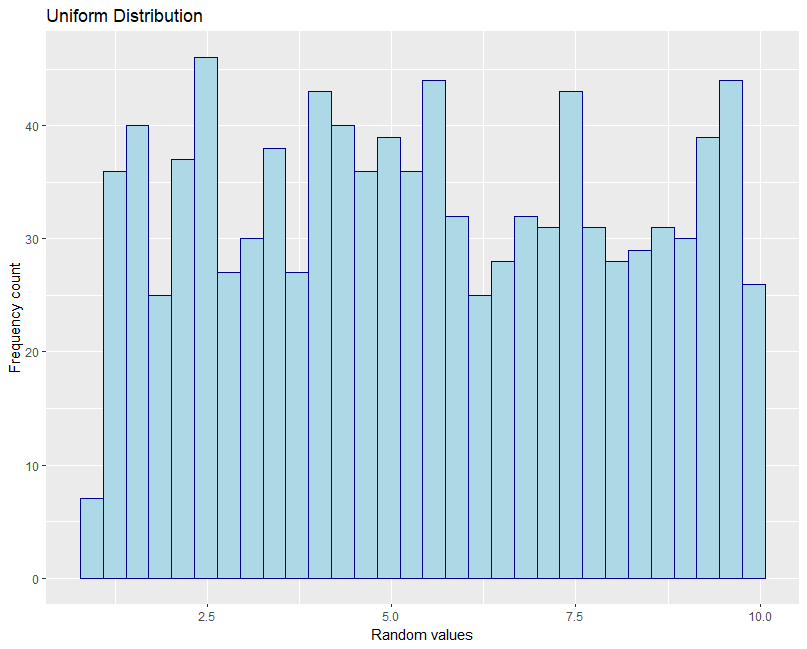# How to Use runif in R (Practical Examples)

`runif()` function from R generates random numbers from uniform distribution.

In uniform distribution, every value within the given range is equally likely to occur. Uniform distribution is mostly used in the generation of random numbers.

The general syntax of `runif()` looks like this:

``````runif(n, min = 0, max = 1)
``````

Where,

Parameter Description
`n` number of random numbers to generate
`min` minimum value for the random numbers (default is 0)
`max` maximum value for the random numbers (default is 1)

The following examples demonstrate how to use the `runif()` (random uniform) function to generate random numbers.

## Example 1: Generate random numbers between 0 and 1

By default, the `runif()` function generates random numbers between 0 to 1 from a uniform distribution.

The following example shows how to generate 10 random numbers between 0 and 1 using `runif()`.

If you run the `runif()` function multiple times, you may see different results every time. To get reproducible random results every time, you should use `set.seed()` function.

``````# for reproducible random result
set.seed(1)

# generate 10 random values between 0 to 1
runif(10)

# output
 0.62911404 0.06178627 0.20597457 0.17655675 0.68702285 0.38410372 0.76984142 0.49769924
 0.71761851 0.99190609
``````

You can see that the 10 random values between 0 and 1 are generated from the uniform distribution.

## Example 2: Generate random numbers between 1 and 100

The following example shows how to generate 10 random numbers between 1 and 100 from uniform distribution using `runif()`.

``````# for reproducible random result
set.seed(1)

# generate 10 random values between 1 to 100
runif(10, 1, 100)

# output
 38.623483 77.967077 93.535818 22.002110 65.515703 13.429955 27.454846 39.225295
  2.325643 38.856408
``````

You can see that the 10 random values between 1 and 100 are generated from the uniform distribution.

## Example 3: Generate random integer numbers between 1 and 100

From the above examples, you have seen that `runif()` function generates floating numbers.

You can use either `round` (gives nearest integer) or `floor` (rounds down to nearest integer) to get the integer values from a uniform distribution

``````# for reproducible random result
set.seed(1)

# generate 10 random integer values between 1 to 100 using round
round(runif(10, 1, 100))

# output
 27 38 58 91 21 90 95 66 63  7

set.seed(1)
# generate 10 random integer values between 1 to 100 using floor
floor(runif(10, 1, 100))

# output
 27 37 57 90 20 89 94 66 63  7
``````

You can see that the 10 random integer values between 1 and 100 are generated from the uniform distribution.

## Example 4: Generate histogram from uniform distribution

You can also `runif()` function to generate random numbers and plot a histogram of uniform distribution (rectangular distribution).

The following example generates 1000 random numbers and plots a histogram,

``````# for reproducible random result
set.seed(1)

# generate 1000 random integer values between 1 to 10 using round
x <- runif(1000, 1, 10)

# Create a histogram to visualize uniform distribution
library(ggplot2)
ggplot() + aes(x)+ geom_histogram(color="darkblue", fill="lightblue") +
xlab("Random values") +
ylab("Frequency count") +
ggtitle("Uniform Distribution")
``````The above histogram visualizes the distribution of 1000 random numbers generated using the `runif()` function.

## Subscribe to get new article to your email when published

* indicates required